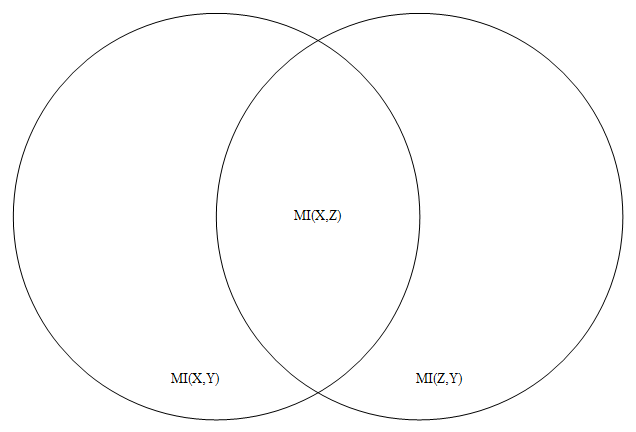# Articles by hundalhh

Mathematician and Father. Into games, astronomy, psychology and philosophy.

## Richardson Extrapolation

Dr Xu at Penn State introduced me to Richardson Extrapolation way back in the early 90’s.  We used it to increase the speed of convergence of finite element analysis algorithms for partial differential equations, but it can be used for many numerical methods.

https://en.wikipedia.org/wiki/Richardson_extrapolation

## Link/Book(pdf): “Regret Analysis of Stochastic and Nonstochastic Multi-armed Bandit Problems”

“Regret Analysis of Stochastic and Nonstochastic Multi-armed Bandit Problems” by Sébastie Bubeck and Nicolò Cesa-Bianchi is available in pdf format at

http://arxiv.org/pdf/1204.5721.pdf

## Newly Published “Deep Learning” Book by Ian Goodfellow, Yoshua Bengio, and Aaron Courville

The book “Deep Learning” by Ian Goodfellow, Yoshua Bengio, and Aaron Courville (associated with the Google Deep Mind Team) is available in HTML format.

http://www.deeplearningbook.org/

## Link: In Two Moves, AlphaGo and Lee Sedol Redefined the Future

Wired has a nice article about the two most brilliant moves in the historic match between AlphaGo and Lee Sedol.

http://www.wired.com/2016/03/two-moves-alphago-lee-sedol-redefined-future/

## Link: AlphaGo Wins against one of the best Go Players on the Planet

In case you had not gotten the news yet, the Go playing program AlphaGo (developed by the Deep Mind division of Google) has beaten Lee Se-dol who is among the top two or three Go players in the world.  Follow the link below for an informative informal video describing AlphaGo and the victory.

Science magazine has a nice pregame report.

http://www.sciencemag.org/news/2016/03/update-why-week-s-man-versus-machine-go-match-doesn-t-matter-and-what-does

## Data Processing Inequality

The Data Processing Inequality is a nice, intuitive inequality about Mutual Information.  Suppose X,Y, Z are random variables and Z is independent of X given Y, then

MI(X,Z) <= MI(X,Y).

See http://www.scholarpedia.org/article/Mutual_information which has an easy one line proof.

We can apply this inequality to a stacked restricted Boltzmann machine (a type of deep neural net).

Let X be a random binary vector consisting of the states of neurons in the first layer.

Let Y be a random binary vector consisting of the states of neurons in the second layer.

And let Z be a random binary vector consisting of the states of neurons in the third layer.

Then

MI(X,Z) <= min( MI(X,Y), MI(Y,Z) ).

Informally, that inequality says that amount of information that can flow from the first layer to the third layer of a stacked RBM deep neural net is less than or equal to the maximum flow rate between the first and second layer.  Also, the amount of information that can flow from the first layer to the third layer is less than or equal to the maximum flow rate between the second and third layer.  This inequality will seem obvious to those who know information theory, but I still think it’s cute.

The above inequality is also sharp in the sense that there are simple examples where the right hand side equals the left hand side.  Consider a Markov Random Field consisting of just three random binary variables X, Y and Z.  Suppose further,  that P(X)=0.5, P(X=Y)=1,  and P(Y=Z)=1.  Then MI(X,Y)=1 bit, MI(Y,Z) =1 bit, and MI(X,Z) =1 bit so both sides of the inequality are 1.

Information theory can also be used to construct a lower bound on the information transfer between the first and third layer.

MI(X,Z) >= MI(Y,X)+MI(Y,Z) – H(Y)

where H(Y) is the entropy of Y (i.e. the information content of the random variable Y).Intuitively, if the sum of the information from X to Y and from Z to Y  exceeds the information capacity of Y, then there must be some information transfer between X and Z.

## A Short Review of “Artificial Neural Networks Based on Fractal Growth”

Zhoa, Li, Geng, and Ma recently wrote a poorly written but interesting paper “Artificial Neural Networks Based on Fractal Growth”.  The paper describes a neural net architecture that grows in a fractal pattern (Similar to evolutionary artificial neural nets, see e.g. “A review of evolutionary artificial neural networks“ Yao 1993).  The input region assigned to each label by the neural net grows in a fractal like pattern to adapt to new data.  The growth of the nodes suggest that the fractal neural network classifications are similar to k-Nearest Neighbor with k=1 or an SVM with radial basis functions.  They report on application of their method to SEMG (Surface electromyogram signal) classification.

## A Short Informal Description of Partially Observable Markov Decision Processes

A partially observable Markov decision process (POMDP) can be informally defined as a world in which an agent can take actions and gain rewards.  The world has a set of possible world states S.  This set can be finite (e.g. mine sweeper) or infinite (e. g. a robotic car in a parking lot).  The world is only partially observable, so if we try to program an agent to act in this world, the robot does not know the state of the entire world, rather it only gets observations that partially reveal the state of the world.  The set of possible observations is typically called Ω.  The agent in a POMDP can take actions.  The actions set is usually called A.  The actions affect the world and result in rewards which also depend on the state of the world.  (Technically, for every world state s and action a there is a reward R(s, a).  R(s, a) is a real number.  Also, for every world state s and action a there is a probability distribution of new possible world states that result after taking action a when the world is in state s.)

## Stanford online course “General Game Playing”

Hey, I just enrolled in Stanford’s General Game Playing CourseGeneral game playing programs are programs that try to play almost any game after being given the rules.  There is a yearly competition of general game playing programs at the AAAI conference.  If you join the course, send me an email so that we can exchange ideas or notes.  (my email address is hundal followed by “hh” at yahoo.com)

Cheers, Hein